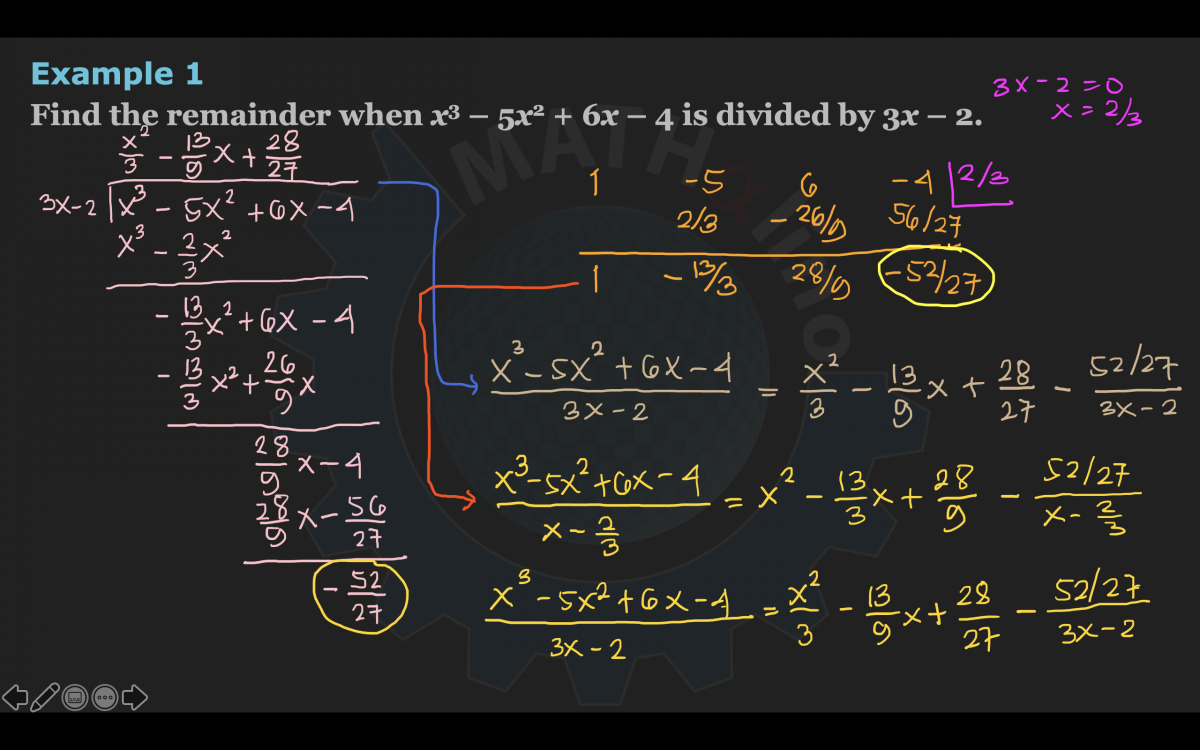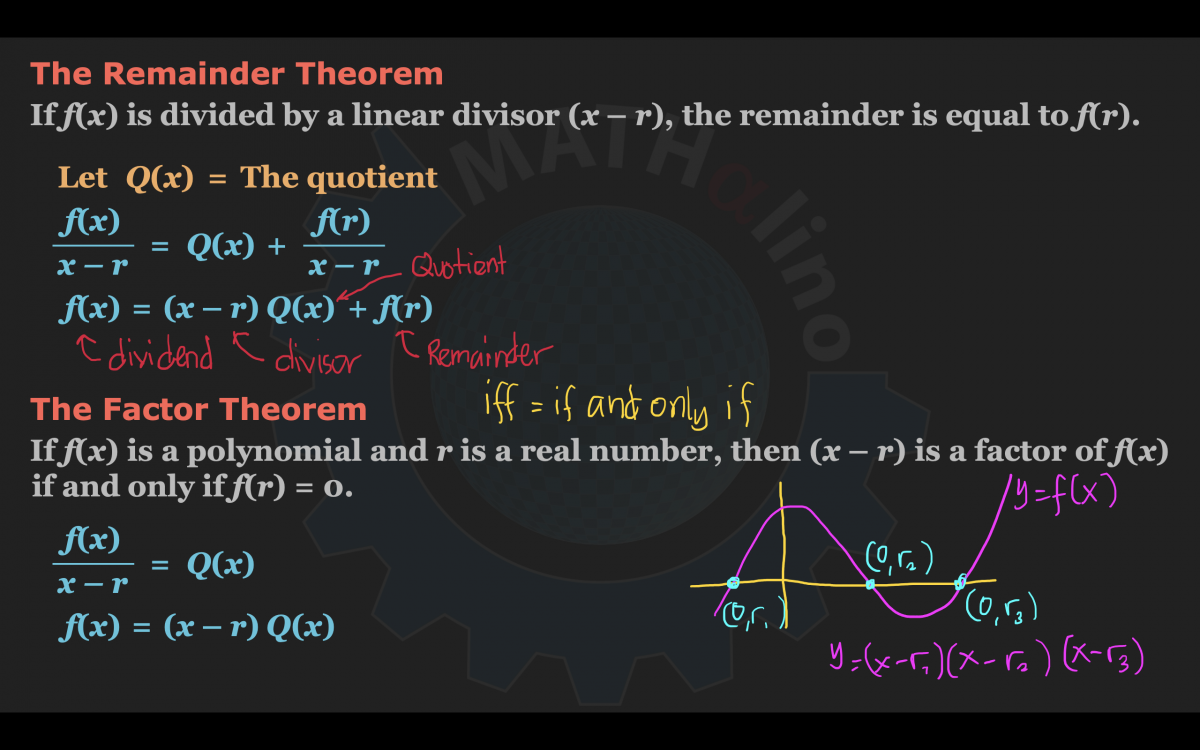# Factor and Remainder Theorems

## Basic Algebra Review Part 7: Factor and Remainder Theorems (Tagalog)

Remainder Theorem
If f(x) is divided by a linear divisor (xr), the remainder is equal to f(r).

Factor Theorem
If f(x) is a polynomial and r is a real number, then (xr) is a factor of f(x) if and only if f(r) = 0.

Mga natakpan ng baby-face...• Mathematics inside the configured delimiters is rendered by MathJax. The default math delimiters are $$...$$ and $...$ for displayed mathematics, and $...$ and $...$ for in-line mathematics.